# Identify Lines of Symmetry (1)

In this worksheet, students must identify the lines of symmetry for the given 2D shapes.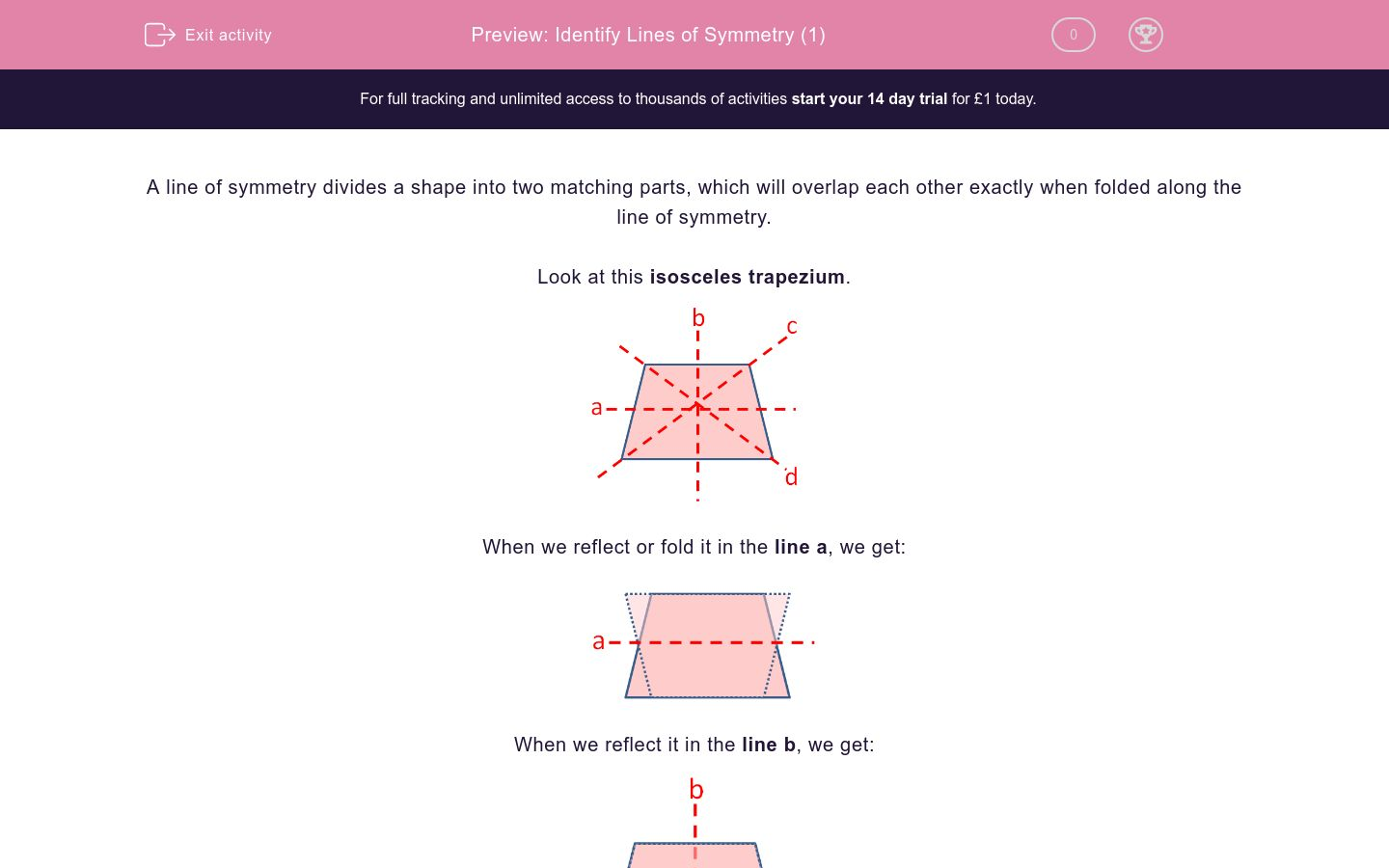Key stage:  KS 2

Curriculum topic:   Maths and Numerical Reasoning

Curriculum subtopic:   Symmetry

Difficulty level:### QUESTION 1 of 10

A line of symmetry divides a shape into two matching parts, which will overlap each other exactly when folded along the line of symmetry.

Look at this isosceles trapezium.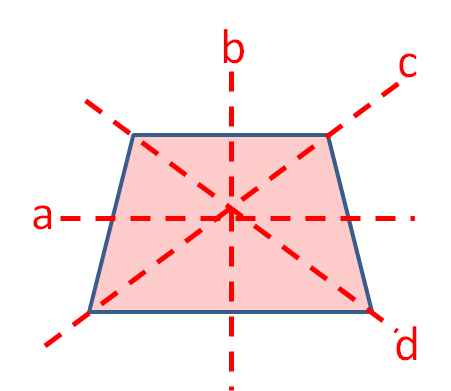When we reflect or fold it in the line a, we get: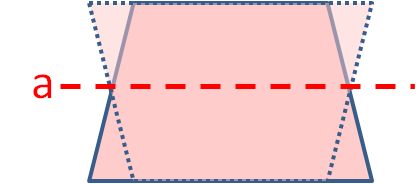When we reflect it in the line b, we get:This is a MATCH!

When we reflect it in the line c, we get:When we reflect it in the line d, we get:It is only in line b that we get a match.

So line b is the only line of symmetry.

Look at this rectangle.

Which of the lines are lines of symmetry?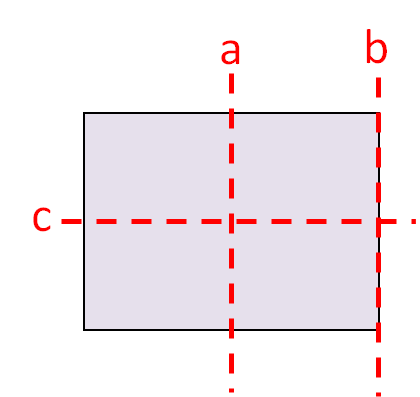a

b

c

Look at this isosceles triangle.

Which of the lines are lines of symmetry?a

b

c

Look at this rectangle.

Which of the lines are lines of symmetry?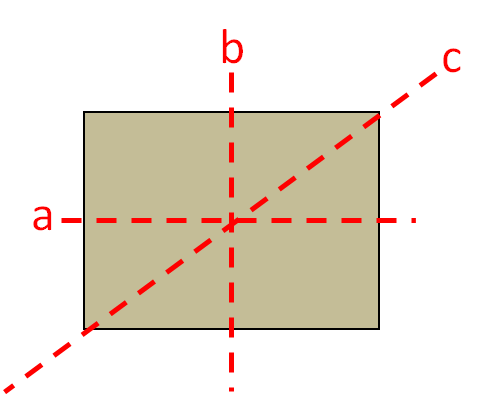a

b

c

Look at this isosceles triangle.

Which of the lines are lines of symmetry?a

b

c

Look at this isosceles triangle.

Which of the lines are lines of symmetry?a

b

c

d

Look at this equilateral triangle.

Which of the lines are lines of symmetry?a

b

c

d

Look at this scalene triangle.

Which of the lines are lines of symmetry?a

b

c

none of these

Look at this square.

Which of the lines are lines of symmetry?a

b

c

d

Look at this parallelogram.

Which of the lines are lines of symmetry?a

b

c

none of these

Look at this square.

Which of the lines are lines of symmetry?a

b

c

d

• Question 1

Look at this rectangle.

Which of the lines are lines of symmetry?a
c
EDDIE SAYS
The two halves of the shape match when folded along lines a and c.
• Question 2

Look at this isosceles triangle.

Which of the lines are lines of symmetry?a
EDDIE SAYS
The two halves of the triangle match when folded along line a.
• Question 3

Look at this rectangle.

Which of the lines are lines of symmetry?a
b
EDDIE SAYS
The two halves of the shape match when folded along lines a and b. The diagonal fold will not give a match.
• Question 4

Look at this isosceles triangle.

Which of the lines are lines of symmetry?c
EDDIE SAYS
Line c is the only fold that will give a match.
• Question 5

Look at this isosceles triangle.

Which of the lines are lines of symmetry?b
EDDIE SAYS
An isosceles triangle only has one line of symmetry. Here it is line b.
• Question 6

Look at this equilateral triangle.

Which of the lines are lines of symmetry?b
c
d
EDDIE SAYS
An equilateral triangle has 3 lines of symmetry from each corner to the middle of the opposite side.
• Question 7

Look at this scalene triangle.

Which of the lines are lines of symmetry?none of these
EDDIE SAYS
Scalene triangles do not have any lines of symmetry.
• Question 8

Look at this square.

Which of the lines are lines of symmetry?a
b
c
d
EDDIE SAYS
A square has 4 lines of symmetry from corner to corner and from the middle of each side to the opposite side.
• Question 9

Look at this parallelogram.

Which of the lines are lines of symmetry?none of these
EDDIE SAYS
Parallelograms do not have any lines of symmetry.
• Question 10

Look at this square.

Which of the lines are lines of symmetry?a
b
c
d
EDDIE SAYS
This is still a square even though it is rotated.
Squares have 4 lines of symmetry.
---- OR ----